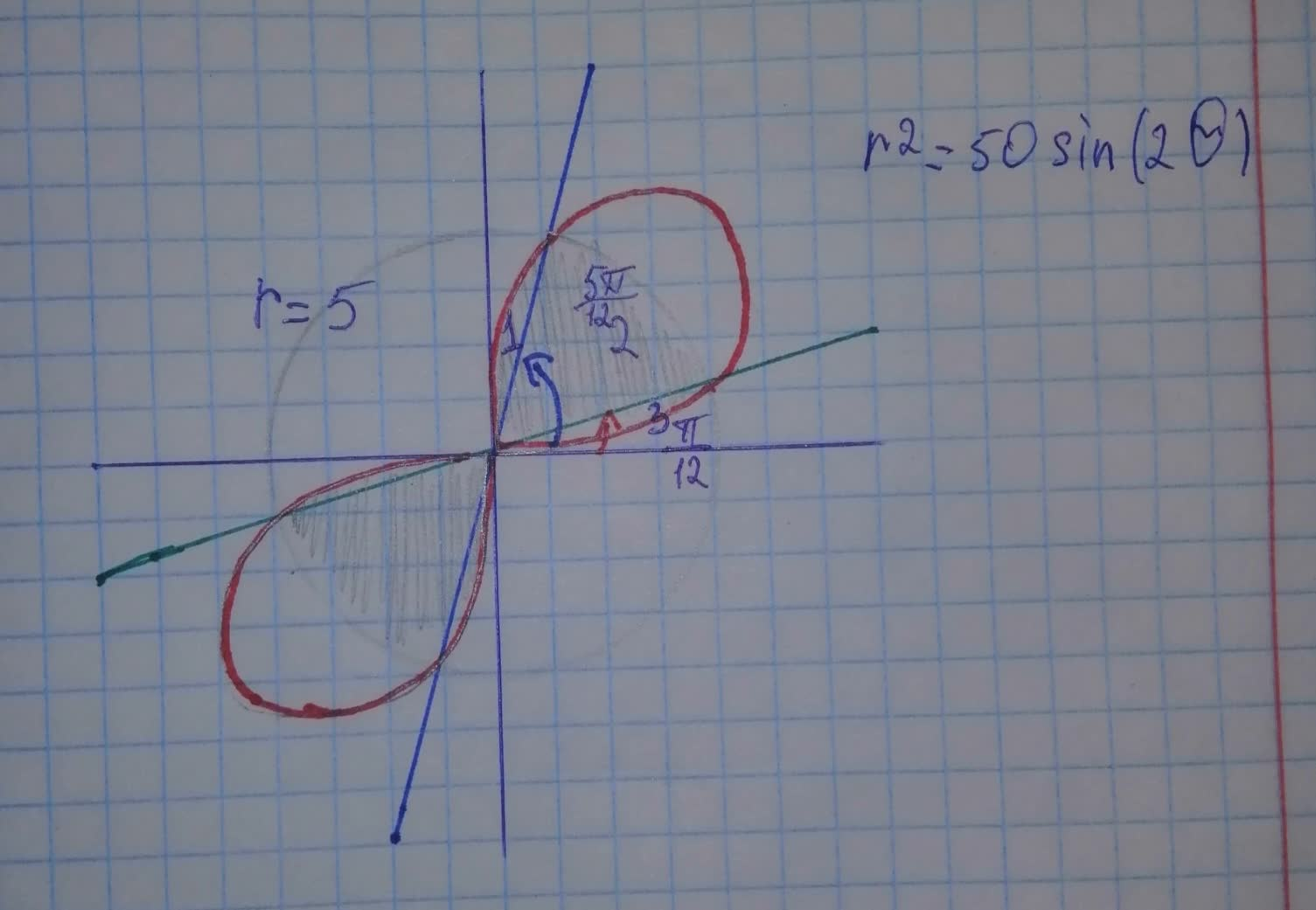# Find the area of the region that lies inside bothAgaiepsh 2021-11-20 Answered
Find the area of the region that lies inside both curves. $$\displaystyle{r}^{{{2}}}={50}{\sin{{\left({2}\theta\right)}}},\ {r}={5}$$

• Questions are typically answered in as fast as 30 minutes

### Plainmath recommends

• Get a detailed answer even on the hardest topics.
• Ask an expert for a step-by-step guidance to learn to do it yourself.Otigh1979
Step 1
Consider the following curves:
$$\displaystyle{r}^{{{2}}}={50}{\sin{{\left({2}\theta\right)}}},\ {r}={5}$$
The objective is to find the area of the region that lies inside both the curves.
Sketch the region as follows:Find the intersection points as follows:
From the curves,
$$\displaystyle{50}{\sin{{2}}}\theta={5}^{{{2}}}$$
$$\displaystyle{\sin{{2}}}\theta={\frac{{{25}}}{{{50}}}}$$
$$\displaystyle{\sin{{2}}}\theta={\frac{{{1}}}{{{2}}}}$$
$$\displaystyle{2}\theta={\frac{{\pi}}{{{6}}}},\ {\frac{{{5}\pi}}{{{6}}}},\ {\frac{{{13}\pi}}{{{6}}}},\ {\frac{{{17}\pi}}{{{6}}}}$$
$$\displaystyle\theta={\frac{{\pi}}{{{12}}}},\ {\frac{{{5}\pi}}{{{12}}}},\ {\frac{{{13}\pi}}{{{12}}}},\ {\frac{{{17}\pi}}{{{12}}}}$$
The formula for the area A of the polar region $$\displaystyle{\mathbb{{{R}}}}$$ is,
$$\displaystyle{A}={\int_{{\alpha}}^{{{b}}}}{\frac{{{1}}}{{{2}}}}{\left[{f{{\left(\theta\right]}}}^{{{2}}}{d}\theta\right.}$$
Step 2
The desired area can be found by adding the area inside the cardioid between $$\displaystyle\theta={0}$$ and $$\displaystyle{\frac{{\pi}}{{{4}}}}$$ from the area inside the circle from 0 to $$\displaystyle{\frac{{\pi}}{{{4}}}}$$
Since the region is symmetric about $$\displaystyle\theta={\frac{{\pi}}{{{4}}}}$$, you can write the area is,
$$\displaystyle{A}={2}{\left\lbrace{2}\times{\frac{{{1}}}{{{2}}}}{\int_{{{0}}}^{{{\frac{{\pi}}{{{12}}}}}}}{\left(\sqrt{{{50}{\sin{{\left({2}\theta\right)}}}}}\right)}^{{{2}}}{d}\theta+{2}\times{\frac{{{1}}}{{{2}}}}{\int_{{{\frac{{\pi}}{{{12}}}}}}^{{{\frac{{\pi}}{{{4}}}}}}}{5}^{{{2}}}{d}\theta\right\rbrace}$$
$$\displaystyle={2}{\left\lbrace{\int_{{{0}}}^{{{\frac{{\pi}}{{{12}}}}}}}{50}{\sin{{\left({2}\theta\right)}}}{d}\theta+{\int_{{{\frac{{\pi}}{{{12}}}}}}^{{{\frac{{\pi}}{{{4}}}}}}}{25}{d}\theta\right\rbrace}$$
$$\displaystyle={2}{\left\lbrace{{\left[-{\frac{{{50}}}{{{2}}}}{\cos{{2}}}\theta\right]}_{{{0}}}^{{{\frac{{\pi}}{{{12}}}}}}}+{25}{{\left[\theta\right]}_{{{\frac{{\pi}}{{{12}}}}}}^{{{\frac{{\pi}}{{{4}}}}}}}\right\rbrace}$$
$$\displaystyle={2}{\left[-{25}{\left({\cos{{\frac{{\pi}}{{{6}}}}}}-{\cos{{0}}}\right)}+{25}{\left({\frac{{\pi}}{{{4}}}}-{\frac{{\pi}}{{{12}}}}\right)}\right]}$$
$$\displaystyle={2}{\left[-{25}{\left({\frac{{\sqrt{{{3}}}}}{{{2}}}}-{1}\right)}+{25}{\left({\frac{{{2}\pi}}{{{12}}}}\right)}\right]}$$
$$\displaystyle={2}{\left(-{\frac{{{25}\sqrt{{{3}}}}}{{{2}}}}+{25}+{\frac{{{25}\pi}}{{{6}}}}\right)}$$
$$\displaystyle=-{25}\sqrt{{{3}}}+{50}+{\frac{{{25}\pi}}{{{3}}}}$$
Thus, the area of the region that lies inside both the curves is
$$\displaystyle-{25}\sqrt{{{3}}}+{50}+{\frac{{{25}\pi}}{{{3}}}}$$